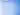# Display Fibonacci series in C ProgramIn this tutorial, learn to display the Fibonacci sequence of first n numbers (entered by the user) in c programming language.

## Fibonacci Series in C using loop

``````#include<stdio.h>
int main()
{
int count, start = 0, second = 1, next, i;

//Ask user to input number of terms
printf("Enter a positive number:\n");
scanf("%d",&count);

printf("First %d terms of Fibonacci series:\n",count);
for ( i = 0 ; i < count ; i++ )
{
if ( i <= 1 )
next = i;
else
{
next = start + second;
start = second;
second = next;
}
printf("%d\n",next);
}

return 0;
}
``````

Output

``````Enter a positive number:
8
First 8 terms of Fibonacci series:
0
1
1
2
3
5
8
13
``````
Tags: